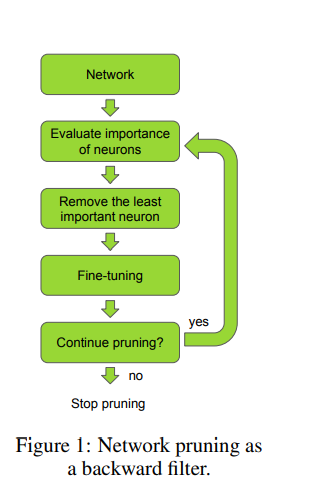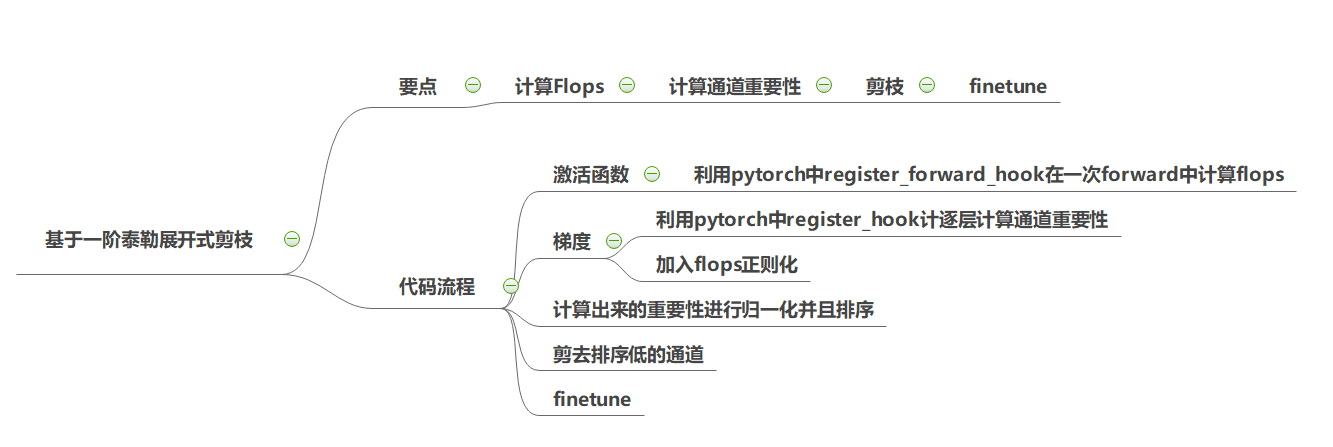# 基于一阶泰勒展开式的结构化剪枝

【GiantPandaCV导语】文章是Nvidia在2017年ICLR发表的，核心思路是将结构化通道剪枝判别依据视为一个优化问题，即最小化剪枝后前后的代价函数之间的差。把优化问题基于一阶泰勒展开式近似，得到通道重要性的判据只需要考虑激活函数与对应的梯度的乘积，再求其均值。为了跨层的公平性加上重要性归一化，以及加入Flops正则化。

## 0.引言¶

1、优化方程是最小化已减去权重的代价函数与未剪枝之前权重的代价函数之差，用一阶泰勒展开式去近似优化方程，计算通道重要性只需要激活函数与对应的梯度的乘积，再求其均值

2、对计算出来的重要性进行$\ell_{2}$归一化；

3、加入FLOPs等正则化，使其硬件更友好；

4、可以联合其他通道重要性判据## 一.剪枝的优化方程¶

$\mathbf{x}$ 表示输入，$y$ 表示输出

\min _{\mathcal{W}^{\prime}}\left|\mathcal{C}\left(\mathcal{D} \mid \mathcal{W}^{\prime}\right)-\mathcal{C}(\mathcal{D} \mid \mathcal{W})\right| \quad

$s.t. \quad\left\|\mathcal{W}^{\prime}\right\|_{0} \leq B$

$\mathbf{z}_{\ell} \in \mathbb{R}^{H_{\ell} \times W_{\ell} \times C_{\ell}}$$z_{\ell}$是一组$H_{\ell} * W_{\ell} * C_{\ell}$特征图， $\ell \in[1, 2, 3,...L]$表示layer

$\mathbf{z}_{\ell}^{(k)}=\mathbf{g}_{\ell}^{(k)} \mathcal{R}\left(\mathbf{z}_{\ell-1} * \mathbf{w}_{\ell}^{(k)}+b_{\ell}^{(k)}\right)$

$\mathbf{g}_{l} \in\{0,1\}^{C}$,表示剪枝与否。

$h_{i}$是由一组参数$\mathcal{W_{i}}$得到的

$\mathcal{C}\left(\mathcal{D} \mid h_{i}\right)=\mathcal{C}\left(\mathcal{D} \mid(\mathbf{w}, b)_{i}\right)$

\left|\Delta \mathcal{C}\left(h_{i}\right)\right|=\left|\mathcal{C}\left(\mathcal{D}, h_{i}=0\right)-\mathcal{C}\left(\mathcal{D}, h_{i}\right)\right|

$\mathcal{C}\left(\mathcal{D}, h_{i}=0\right)$表示被剪去参数的代价函数 $\mathcal{C}\left(\mathcal{D}, h_{i}\right)$是原来参数的代价函数

\mathcal{C}\left(\mathcal{D}, h_{i}=0\right)=\mathcal{C}\left(\mathcal{D}, h_{i}\right)-\frac{\delta \mathcal{C}}{\delta h_{i}} h_{i}+R_{1}\left(h_{i}=0\right)

R_{n}(x)=f^{(n+1)}\left[x_{0}+\theta\left(x-x_{0}\right)\right] \frac{\left(x-x_{0}\right)^{n+1}}{(n+1) !}

R_{1}\left(h_{i}=0\right)=\frac{\delta^{2} \mathcal{C}}{\delta\left(h_{i}^{2}=\xi\right)} \frac{h_{i}^{2}}{2}, \xi\in(0,h_{i})

\Theta_{T E}\left(h_{i}\right)=\left|\Delta \mathcal{C}\left(h_{i}\right)\right|=\left|\mathcal{C}\left(\mathcal{D}, h_{i}\right)-\frac{\delta \mathcal{C}}{\delta h_{i}} h_{i}-\mathcal{C}\left(\mathcal{D}, h_{i}\right)\right|=\left|\frac{\delta \mathcal{C}}{\delta h_{i}} h_{i}\right|

\Theta_{T E}\left(z_{l}^{(k)}\right)=\left|\frac{1}{M} \sum_{m} \frac{\delta C}{\delta z_{l, m}^{(k)}} z_{l, m}^{(k)}\right|

\hat{\Theta}\left(\mathbf{z}_{l}^{(k)}\right)=\frac{\Theta\left(\mathbf{z}_{l}^{(k)}\right)}{\sqrt{\sum_{j}\left(\Theta\left(\mathbf{z}_{l}^{(j)}\right)\right)^{2}}}

\Theta\left(\mathbf{z}_{l}^{(k)}\right)=\Theta\left(\mathbf{z}_{l}^{(k)}\right)-\lambda \Theta_{l}^{f l o p s}

## 二、核心代码实现¶1、通道重要性计算

def compute_rank(self, grad, lamda=1):
activation_index = len(self.activations) - self.grad_index - 1
activation = self.activations[activation_index]

taylor = activation * grad                  ##计算基于一阶泰勒展开的值
taylor = taylor.mean(dim=(0, 2, 3)).data    ## 以通道维度计算得分

flops = self.flops_dict[activation_index] * torch.ones_like(taylor) ###FLOPs 正则化,见下面get_flops()
taylor = taylor - lamda * flops # 公式(8)

if activation_index not in self.filter_ranks:
self.filter_ranks[activation_index] = torch.FloatTensor(activation.size(1)).zero_().cuda()

self.filter_ranks[activation_index] += taylor


2、计算FLOPs

def get_flops(self):
self.flops_dict = {}
def hook(module, inputs, outputs):  #hook函数，用来计算每层的flops
params = module.weight.size().numel()  #torch.numel() 返回一个tensor变量内所有元素个数，可以理解为矩阵内元素的个数
W = outputs.size(2)
H = outputs.size(3)
module.flops = params * W * H   #计算flops

for m in model.modules():
if isinstance(m, nn.Conv2d):
m.register_forward_hook(hook) #为每一层注册hook
x = torch.randn(1, 3, 224, 224) #随便定义一个数据，跑一次forward来获取flops
_ = self.model(x)
activation_index = 0
for m in self.model.modules():
if isinstance(m, nn.Conv2d):
self.flops_dict[activation_index] = m.flops  #获取每层的flops，记录到字典
activation_index += 1


3、对基于一阶泰勒展开式计算得到重要性，进行通道归一化

def normalize_ranks_per_layer(self):
for i in self.filter_ranks:
v = torch.abs(self.filter_ranks[i])
v = v / torch.sqrt(torch.sum(v * v))
self.filter_ranks[i] = v


## 三、文章的一些讨论¶

1、泰勒展开的讨论

1990年 LeCun提出Optimal Brain Damage (OBD) 也是采用泰勒展开式的方法来近似。因为经过多次训练迭代后，梯度会趋向于0，那么$y=\frac{\delta \mathcal{C}}{\delta h} h$，则$\mathbb{E}(y)=0$，所以LeCun认为$y$提供的信息很有限，$\mathcal{C}\left(\mathcal{D}, h_{i}\right)-\frac{\delta \mathcal{C}}{\delta h_{i}} h_{i}-\mathcal{C}\left(\mathcal{D}, h_{i}\right) =0$ ，所以采用泰勒二阶展开式。

2、通道重要性

## 四、参考链接¶